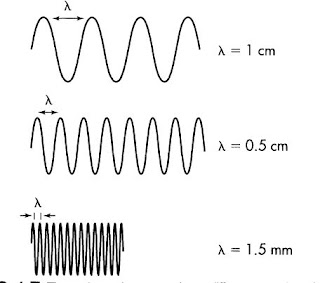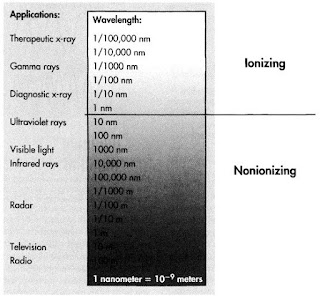# Electromagnetic Energy Xray Production

## Saturday, July 21, 2018

#### Electromagnetic Energy

Xrays are among several types of energy described as electromagnetic energy, or electromagnetic wave radiation. They have both electrical and magnetic properties, changing the field through which they pass both electrically and magnetically. These changes in the field occur in the form of a repeating wave, a pattern that scientists call a sinusoidal form or sine wave.
Several types of this waveform are significant. The distance between the crest and valley of the wave (it’s height) is called the amplitude.The three sine waves are identical except for their amplitudes.

More important to the radiographer is the distance from one crest to the next or wavelength.Three sine waves have different wavelengths. The shorter the wavelength, the higher the frequency.

The frequency of the waves is the number of times per seconds that a crest passes a given point.

Because all electromagnetic energy moves through space at the same velocity, at approximately 186,000 miles per seconds, which is 30 billion (3 x 1010) centimetre per second, it is apparent that a relationship exist between wavelength and frequency. When the wavelength is short, the crest are closer together, so more of them will pass a given point each second, resulting in a higher frequency. Longer wavelengths will have a lower frequency. This may be expressed mathematically as follows:

Velocity (v) = Wavelength (λ) x Frequency (f)

The more energy the wave has, the greater will be its frequency and the shorter its wavelength. We can therefore use either wavelength or frequency to describe the energy of the wave. In radiologic science, wavelength is more often used to describe the energy of the xray beam. The average wavelength of a diagnostic xray beam is approximately 0.1 nanometer, which is 10-10 (0.00000000001) meters, or about a billionth of an inch.

The wavelength of electromagnetic radiation varies from exceedingly short ( shorter than that of diagnostic xrays) to very long ( more than 5 miles). This range of energies is known as the electromagnetic spectrum. It includes xray, gamma rays, visible light, microwaves, and radio waves.Electromagnetic Spectrum

Radiation with a wavelength shorter than one nanometer (10-9 meters) is said to be ionizing radiation because it has sufficient energy to remove an electron from an atomic orbit. Xray are one type of ionizing radiation.

The smallest possible unit of electromagnetic energy (analogous to the atom with respect to matter) is the photon, which may be thought of as a minute “bullet” of energy. Photons occur in group or “bundles” called quanta or quantum (singular).